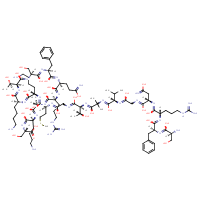Structure InformationCompound Identification

SMILES

CSCC[C@H](NC(=O)CNC(=O)[C@@H](NC(=O)C(C)(C)NC(=O)[C@@H](NC(=O)CNC(=O)[C@H](CC(N)=O)NC(=O)[C@H](CCCNC(N)=N)NC(=O)[C@H](CC1=CC=CC=C1)NC(=O)[C@@H](N)CO)C(C)C)[C@@H](C)O)C(=O)N[C@@H](CCCCN)C(=O)N[C@@H](CCCCN)C(=O)N[C@@H]([C@@H](C)O)C(=O)N[C@@H](CO)C(=O)N[C@@H](CC1=CC=CC=C1)C(=O)N[C@@H](CCC(N)=O)C(=O)N[C@@H](CCCNC(N)=N)C(=O)N[C@@H](C)C(=O)N[C@@H](CCCCN)C(=O)N[C@@H](CO)C(O)=O

InChIKey

InChIKey=ACOVNTWZIJRSIM-OHOMDISFSA-N

Formula

C95H159N31O28S

Mass

2215.57

### Export to:

Taxonomic Classification

Taxonomy Tree

Kingdom

Organic compounds

Superclass

Organic Polymers

Class

Polypeptides

Subclass

Not available

Intermediate Tree Nodes

Not available

Direct Parent

Polypeptides

Alternative Parents

Molecular Framework

Aromatic homomonocyclic compounds

Substituents

Polypeptide - Alpha peptide - Arginine or derivatives - Phenylalanine or derivatives - Glutamine or derivatives - Methionine or derivatives - Asparagine or derivatives - N-acyl-alpha amino acid or derivatives - Valine or derivatives - N-acyl-l-alpha-amino acid - N-acyl-alpha-amino acid - Alpha-amino acid amide - Serine or derivatives - Alanine or derivatives - N-substituted-alpha-amino acid - Amphetamine or derivatives - Alpha-amino acid or derivatives - Beta-hydroxy acid - Monocyclic benzene moiety - Fatty amide - Hydroxy acid - Benzenoid - N-acyl-amine - Fatty acyl - Amino acid or derivatives - Amino acid - Secondary carboxylic acid amide - Secondary alcohol - Primary carboxylic acid amide - Carboxamide group - Guanidine - Dialkylthioether - Sulfenyl compound - Carboximidamide - Thioether - Carboxylic acid derivative - Carboxylic acid - Monocarboxylic acid or derivatives - Primary alcohol - Organic oxygen compound - Amine - Primary amine - Carbonyl group - Organic oxide - Alcohol - Organic nitrogen compound - Organosulfur compound - Imine - Primary aliphatic amine - Hydrocarbon derivative - Organooxygen compound - Organonitrogen compound - Aromatic homomonocyclic compound

Description

This compound belongs to the class of organic compounds known as polypeptides. These are peptides containing ten or more amino acid residues.

External Descriptors

Not available